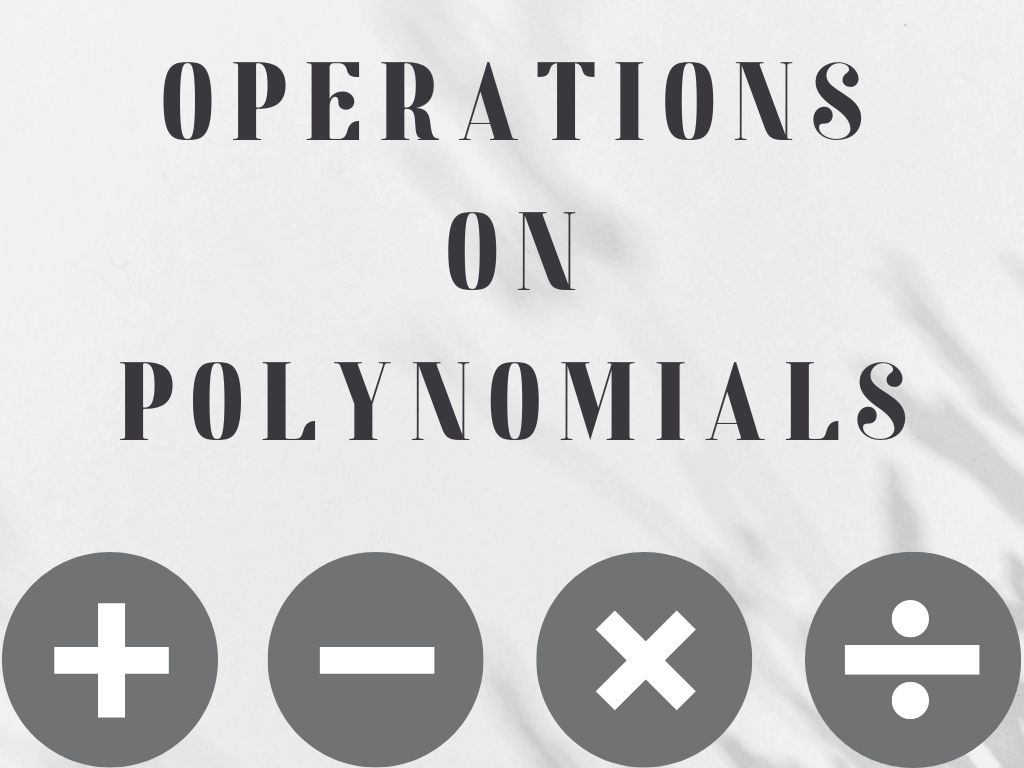# Operations on Polynomials Class 10th

## Introduction

Operations on polynomials are the addition of polynomials, the subtraction of polynomials, the multiplication of polynomials, and the division of polynomials.

➤ Subtraction of polynomials

➤ Multiplication of polynomials

➤ Division of polynomials

When we add two or more polynomials then only like terms are added. it means terms with the same variables and powers are added. unlike terms will not be added, they will remain unchanged. In addition, the degree of the resultant polynomial remains the same.

Example – Add polynomials 3x2 + 4xy + 2y2 and 5y2 – xy + 7x2

Solution – 3x2 + 4xy + 2y2 + 5y2 – xy + 7x2

(3x2 + 7x2) + (4xy – xy) + (2y2 + 5y2)

10x2 + 3xy + 7y2      Ans.

### Subtraction of Polynomials

Subtraction of the polynomials is the same as the addition of the polynomials. In this, like terms are subtracted and the unlike terms will be unchanged. In this also, the degree of the resultant polynomial will remain the same.

Example – Subtract the polynomial 3xy2 + 4xy – 6x2y + y3 from 8xy2 + 7xy + x2y.

Solution – 8xy2 + 7xy + x2y – (3xy2 + 4xy – 6x2y + y3)

8xy2 + 7xy + x2y – 3xy2 – 4xy + 6x2y – y3

(8xy2 – 3xy2) + (7xy – 4xy) + (x2y + 6x2y) – y3

5xy2 + 3xy + 7x2y – y3        Ans.

### Multiplication of Polynomials

When two or more polynomials are multiplied then the result is always of a higher degree polynomial. But in two polynomials, if one or both of the polynomials are constant polynomials then the degree will remain the same. In the multiplication of polynomials, powers of the same variables are added by the laws of exponents.

#### Multiplying a Monomial by a Monomial

Multiplying two monomials

Examples – 1) 4x⨯6y = (4⨯6)⨯(x⨯y) = 24xy

2) 3a⨯(-2b) = {3⨯(-2)}⨯(a⨯b) = -6ab

3) 6p2⨯8p = (6⨯8)⨯(p2⨯p) = 48p3

4) x4yz2⨯2xy3z = (1⨯2)⨯(x4⨯x⨯y⨯y3⨯z2⨯z) = 2x5y4z3 Ans.

Multiplying three or more monomials

Examples – 1) 7t⨯2s⨯3r = (7⨯2⨯3)⨯(t⨯s⨯r) = 42tsr Ans.

2) 4pq⨯5p2q2⨯6p3q3

= (4⨯5⨯6)⨯(p⨯p2⨯p3⨯q⨯q2⨯q3)

= 120p6q6 Ans.

3) 2x2y⨯(-4y2z)⨯(-7z2x)⨯2x2yz

= [2x2y⨯(-4y2z)]⨯[(-7z2x)⨯2x2yz]

= (-8x2y3z)⨯(-14x3yz3)

= 112x5y4z4 Ans.

#### Multiplying a Monomial by a Polynomial

Multiplying a monomial by a binomial

Examples – 1) 2a⨯(3b+4)

Using Distributive law,

= 2a⨯3b + 2a⨯4

= 6ab + 8a     Ans.

2) 7xy⨯(y2+5)

= 7xy⨯y2 + 7xy⨯5

= 7xy3 + 35xy Ans.

Multiplying a monomial by a trinomial

Examples – 1) 3v⨯(4v2+5v+7)

Using Distributive law,

= 3v⨯4v2 + 3v⨯5v + 3v⨯7

= 12v3 + 15v2 + 21v Ans.

2) x2⨯(9xz+6y2-xyz)

= x2⨯9xz + x2⨯6y2 – x2⨯xyz

= 9x3z + 6x2y2 – x3yz Ans.

#### Multiplying a Polynomial by a Polynomial

Multiplying a binomial by a binomial

Examples – 1) (2x+3y)⨯(3x+2y)

= 2x⨯(3x+2y) + 3y⨯(3x+2y)

= 2x⨯3x + 2x⨯2y + 3y⨯3x + 3y⨯2y

= 6x2 + 4xy + 9xy + 6y2

= 6x2 + 13xy + 6y2 Ans.

2) (p2-6qr)⨯(9q2+7r)

= p2⨯(9q2+7r) – 6qr⨯(9q2+7r)

= p2⨯9q2 + p2⨯7r – 6qr⨯9q2 – 6qr⨯7r

= 9p2q2 + 7p2r – 54q3r – 42qr2 Ans.

Multiplying a binomial by a trinomial

Examples – 1) (a+5)⨯(a2+2a-7)

= a⨯(a2+2a-7) + 5⨯(a2+2a-7)

= a⨯a2+a⨯2a-a⨯7 + 5⨯a2+5⨯2a-5⨯7

= a3+2a2-7a + 5a2+10a-35

= a3+7a2+3a-35 Ans.

2) (x2-3)⨯(xy+yz+zx)

= x2⨯(xy+yz+zx) – 3⨯(xy+yz+zx)

= x2⨯xy+x2⨯yz+x2⨯zx – 3⨯xy – 3⨯yz – 3⨯zx

= x3y + x2yz + x3z – 3xy – 3yz – 3zx Ans.

### Division of Polynomials

In the division of polynomials, the result is a lesser degree polynomial and if one of the polynomials is a constant polynomial then the degree will remain the same.

#### Dividing a Monomial by a Monomial

For easy Division, we factorize both Dividend and Divisor in this type of Division.

Examples – 1) 6x4 ÷ 3x

= 2⨯3⨯x⨯x⨯x⨯x/3⨯x = 2⨯x⨯x⨯x = 2x3 Ans.

2) 72p2q3r ÷ (- 6p4q)

= 2⨯2⨯2⨯3⨯3⨯p⨯p⨯q⨯q⨯q⨯r/-2⨯3⨯p⨯p⨯p⨯p⨯q

= 2⨯2⨯3⨯q⨯q⨯r/-p⨯p = 12q2r/-p2 Ans.

#### Dividing a Polynomial by a Monomial

Examples – 1) (2xy + 6x) ÷ 2x

= (2xy + 6x)/2x = 2x(y + 3)/2x = (y+3) Ans.

2) (8y3 + 6y2 + 12y) ÷ 2y

= (8y3 + 6y2 + 12y)/2y = 2y(4y2 + 3y+ 6)/2y

=  4y2 + 3y+ 6 Ans.

This can be also solved as = 8y3/2y + 6y2/2y + 12y/2y = 4y2 + 3y+ 6

#### Dividing a Polynomial by a Polynomial

Examples – 1) (7a2 + 14a) ÷ (a + 2)

= (7a2 + 14a)/(a + 2) = 7a(a + 2)/(a + 2) = 7a Ans.

2) (x4 – 5x3 – 24x2) ÷ x(x – 8)

= (x4 – 5x3 – 24x2)/x(x – 8) = x2(x2 – 5x – 24)/x(x – 8)

= x(x2 – 8x + 3x – 24)/(x – 8)   [factorization of (x2 – 5x – 24)]

= x{x(x – 8) + 3(x – 8)}/(x – 8)

x(x – 8)(x + 3)/(x – 8) = x(x + 3)      Ans.

If the above method does not help you to divide a polynomial by a polynomial, then there is also another method to divide which is called the Division Algorithm.

Operations on Polynomials Class 10th in Hindi